# Tuple

(diff) ← Older revision | Latest revision (diff) | Newer revision → (diff)
A finite sequence (admitting repetitions) of elements from some set. A tuple is denoted by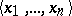,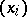,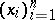,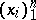,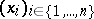,, or. The numberis called its length (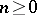),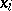is called the-th term of the-tuple and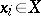(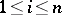). For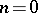one finds the empty tuple, which contains no terms.
Synonyms of the term tuple are the following: a word in the alphabet(in this case it is usually assumed thatis finite); an element of some Cartesian power of the set; an element of the free semi-group with identity generated by; a function defined on the firstnatural numbers () with values in.
The typical property of tuples is that a tuple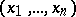is equal to another one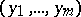precisely whenand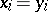for all. In the context of a set-theoretical foundation of mathematics (such as Zermelo–Fraenkel set theory), where every object must be a set or a class, tuples are usually constructed as sets by the following inductive procedure:is the empty set for, and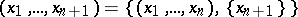.